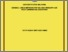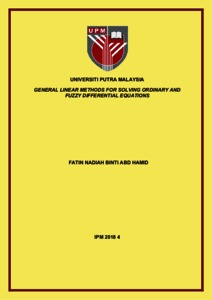Simple Search:

General linear methods for solving ordinary and fuzzy differential equations

Citation

Abd Hamid, Fatin Nadiah (2017) General linear methods for solving ordinary and fuzzy differential equations. Masters thesis, Universiti Putra Malaysia.

Abstract / Synopsis

In this thesis, a third order General Linear Method (GLM) is proposed for solving ordinary and fuzzy differential equations as well as second kind of fuzzy Volterra integro-differential equations (FVIDEs). Also, the fuzzy version of Improved Runge-Kutta (IRK) method is adapted to solve the second kind of FVIDEs. Third order General Linear Method is derived using the technique of rooted trees and B-series. The algebraic order conditions of the method are obtained up to trees of order four. Using the order conditions, we obtained the different sets of coefficients for GLM of order three. Stability region is discussed and numerical results of GLMs for solving first order ordinary differential equations (ODEs) are compared with the existing method. Subsequently, numerical solutions of first order fuzzy differential equations (FDEs) are proposed using fuzzy version of obtained GLM. The approach of generalized Hukuhara differentiability is used to define the FDEs. Based on this approach, the characterization theorem which converted the FDEs into systems of ODEs is explored. Then, a fuzzy version of third order GLM for solving FDEs using the generalized Hukuhara differentiability is developed. The convergence of the method is given and numerical results compared with different existing methods are presented.The study of FDEs is then extended to the first order fuzzy Volterra integrodifferential equations. Unlike FDEs, a differential and integral operators appear simultaneously in FVIDEs. Therefore, suitable numerical quadrature rules which are the combination of composite Simpson’s rule together with Lagrange interpolation polynomial and Trapezoidal rule are used to solve the integral part whereas the third order GLM is considered for the differential part. Again, the generalized Hukuhara differentiability is applied to develop the GLM combined with given numerical quadrature rules for solving FVIDEs. Numerical results are tabulated to illustrate the performance of the proposed method. Finally, Improved Runge-Kutta method of order four with three stages is proposed to obtain the numerical solutions of FVIDEs. A similar strategy is used to develop the Improved Runge-Kutta method by adapting the same numerical quadrature rules as used for General Linear Method and is based on generalized Hukuhara differentiability. The performance of Improved Runge-Kutta method is demonstrated by comparing the numerical results with the existing method of same order.Preview
PDF
IPM 2018 4 IR.pdfView Item